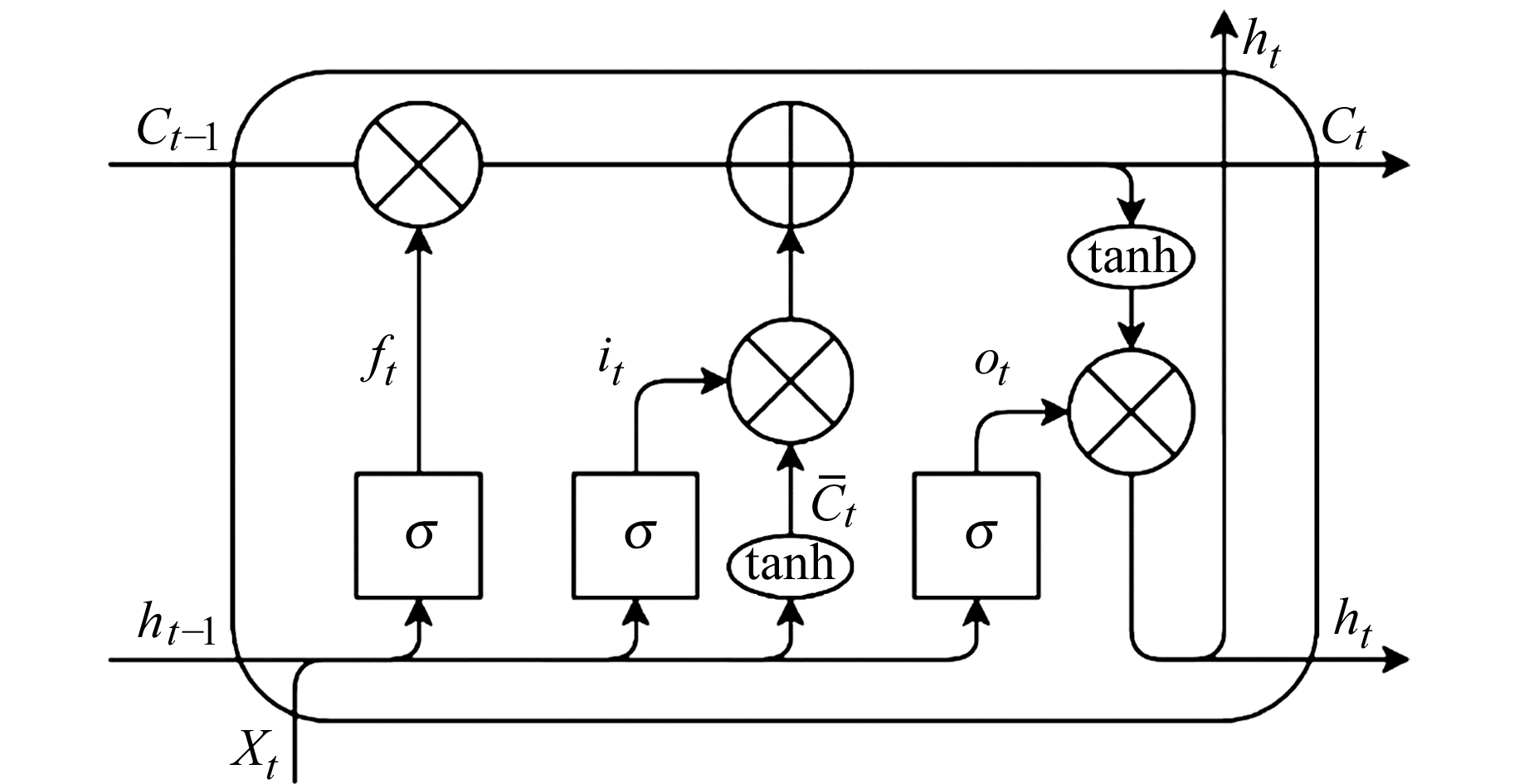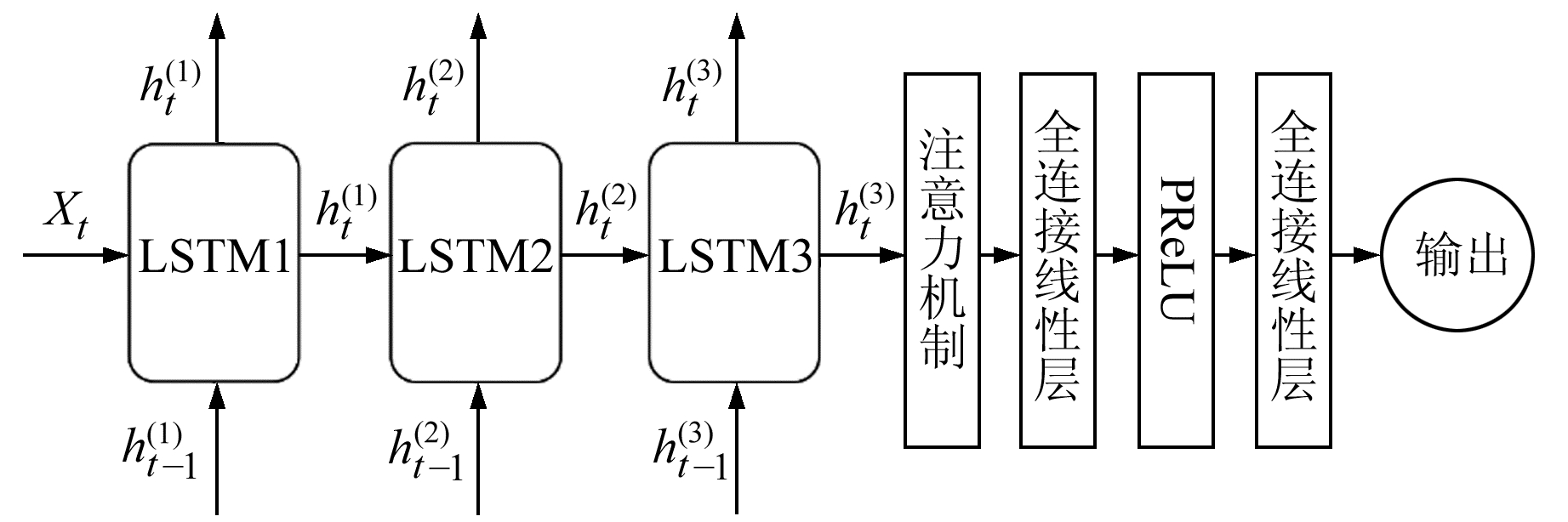﻿ 基于深度神经网络的GRACE等效水高及地表形变量预测文章快速检索 高级检索
 大地测量与地球动力学2021, Vol. 41Issue (7): 721-726  DOI: 10.14075/j.jgg.2021.07.011### 引用本文YAO Zhiwei, CHEN Yu. Time Series Forecasting of Equivalent Water Height and Surface Displacements from GRACE Using Deep Neural Networks[J]. Journal of Geodesy and Geodynamics, 2021, 41(7): 721-726.### Foundation support

Social Science Research Project of Sichuan University, No. SKBM201915.

### Corresponding author

CHEN Yu, PhD, associate professor, majors in remote sensing images analysis and water conservancy informatization, E-mail: ychen@scu.edu.cn.

### 第一作者简介

YAO Zhiwei, postgraduate, majors in deep learning in time series prediction, E-mail: yzw_job@163.com.

### 文章历史

1. 四川大学电子信息学院，成都市一环路南一段24号，610065

1 GRACE数据处理

2 模型搭建 2.1 LSTM网络基本原理图 1 LSTM结构 Fig. 1 LSTM structure

LSTM结构包含遗忘门ft、输入门it和输出门otft决定上一时刻细胞状态值Ct-1被遗忘的程度，it则决定记住哪些信息，当前细胞状态值Ct的更新需要ftit的共同作用，输出层ot根据细胞状态Ct以及输入值Xt来决定最终的输出值ht。每层的输出如下：

 ${f_t} = \sigma ({W_f} \cdot [{h_{t - 1}}, {X_t}] + {b_f})$ (1)
 ${i_t} = \sigma ({W_i} \cdot [{h_{t - 1}}, {X_t}] + {b_i})$ (2)
 ${{\bar C}_t} = {\rm{tanh}}({W_C} \cdot [{h_{t - 1}}, {X_t}] + {b_C})$ (3)
 ${C_t} = {f_t} \otimes {C_{t - 1}} \otimes {i_t} \otimes {{\bar C}_t}$ (4)
 ${o_t} = \sigma ({W_o} \cdot [{h_{t - 1}}, {X_t}] + {b_o})$ (5)
 ${h_t} = {o_t} \otimes {\rm{tanh}}({C_t})$ (6)

2.2 深度LSTM网络图 2 深度LSTM网络结构 Fig. 2 Deep LSTM network structure

3 模型训练 3.1 训练超参数设置表 1 深度LSTM结构及超参数 Tab. 1 Deep LSTM structure and hyper parameters
3.2 训练数据处理

4 结果分析 4.1 模型评价指标 4.1.1 均方根误差RMSE

 ${\rm{RMSE}} = \sqrt {\frac{1}{n}\sum\limits_{i = 1}^n ( {x_i} - {y_i}{)^2}}$ (7)

 ${R^*} = \frac{{{\rm{RMSE}}}}{{\sqrt {{\rm{var}}(y)} }}$ (8)
4.1.2 纳什系数NSE

NSE系数可以表示模型相对于实际数据平均值的预测能力，可表明实际值与模型预测数据曲线是否符合1 ∶1的线性关系。NSE被认为是评价预测值与观测值整体拟合情况的最佳目标函数，可用于对比不同模型对同一数据的拟合优度。NSE系数最佳值为1.0，计算公式为：

 ${\rm{NSE}} = 1 - \frac{{\sum\limits_{i = 1}^n {{{({x_i} - {y_i})}^2}} }}{{\sum\limits_{i = 1}^n {{{({y_i} - \bar y)}^2}} }}$ (9)

4.2 结果分析表 2 动态模式 Tab. 2 Dynamic mode表 3 静态模式 Tab. 3 Static mode图 3 三峡区域预测结果 Fig. 3 Prediction results in the Three Gorges region
5 结语

  宁津生. 卫星重力探测技术与地球重力场研究[J]. 大地测量与地球动力学, 2002, 22(1): 1-5 (Ning Jinsheng. The Satellite Gravity Surveying Technology and Research of Earth's Gravity Field[J]. Journal of Geodesy and Geodynamics, 2002, 22(1): 1-5) (0)  郑伟, 许厚泽, 钟敏, 等. 地球重力场模型研究进展和现状[J]. 大地测量与地球动力学, 2010, 30(4): 83-91 (Zheng Wei, Xu Houze, Zhong Min, et al. Progress and Present Status of Research on Earth's Gravitational Field Models[J]. Journal of Geodesy and Geodynamics, 2010, 30(4): 83-91) (0)  Frappart F, Papa F, Silva J S, et al. Surface Freshwater Storage and Dynamics in the Amazon Basin during the 2005 Exceptional Drought[J]. Environmental Research Letters, 2012, 7(4): 44 010 DOI:10.1088/1748-9326/7/4/044010 (0)  Li S Y, Shen W B, Pan Y J, et al. Surface Seasonal Mass Changes and Vertical Crustal Deformation in North China from GPS and GRACE Measurements[J]. Geodesy and Geodynamics, 2020, 11(1): 46-55 DOI:10.1016/j.geog.2019.05.002 (0)  赵少荣, 刘庆元, 朱建军. 联合GPS和GRACE卫星数据反演北美洲的冰川均衡调整模式[J]. 大地测量与地球动力学, 2013, 33(3): 34-40 (Zhao Shaorong, Liu Qingyuan, Zhu Jianjun. Glacial Isostatic Adjustment Models Constrained by Joint Inversion of GPS and GRACE Satellite Observations in North America[J]. Journal of Geodesy and Geodynamics, 2013, 33(3): 34-40) (0)  Bengio Y, Simard P, Frasconi P. Learning Long-Term Dependencies with Gradient Descent is Difficult[J]. IEEE Transactions on Neural Networks, 1994, 5(2): 157-166 DOI:10.1109/72.279181 (0)  Hochreiter S, Schmidhuber J. Long Short-Term Memory[J]. Neural Computation, 1997, 9(8): 1 735-1 780 DOI:10.1162/neco.1997.9.8.1735 (0)  孙宇航, 刘洋. 利用GRU神经网络预测横波速度[J]. 石油地球物理勘探, 2020, 55(3): 484-492 (Sun Yuhang, Liu Yang. Prediction of S-Wave Velocity Based on GRU Neural Network[J]. Oil Geophysical Prospecting, 2020, 55(3): 484-492) (0)  赵勇, 苏丹, 邹丽, 等. 基于LSTM神经网络的畸形波预测[J]. 华中科技大学学报: 自然科学版, 2020, 48(7): 47-51 (Zhao Yong, Su Dan, Zou Li, et al. Rogue Wave Prediction Based on LSTM Neural Network[J]. Journal of Huazhong University of Science and Technology: Natural Science Edition, 2020, 48(7): 47-51) (0)  Shi X J, Chen Z R, Wang H, et al. Convolutional LSTM Network: A Machine Learning Approach for Precipitation Nowcasting[M]//Cortes C, Lawrence N D, Lee D D, et al. Advances in Neural Information Processing Systems 28. Cambridge: MIT Press, 2015 (0)  张建海, 张棋, 许德合, 等. ARIMA-LSTM组合模型在基于SPI干旱预测中的应用——以青海省为例[J]. 干旱区地理, 2020, 43(4): 1 004-1 013 (Zhang Jianhai, Zhang Qi, Xu Dehe, et al. Application of a Combined ARIMA-LSTM Model Based on SPI for the Forecast of Drought: A Case Study in Qinghai Province[J]. Arid Land Geography, 2020, 43(4): 1 004-1 013) (0)  殷兆凯, 廖卫红, 王若佳, 等. 基于长短时记忆神经网络(LSTM)的降雨径流模拟及预报[J]. 南水北调与水利科技, 2019, 17(6): 1-9 (Yin Zhaokai, Liao Weihong, Wang Ruojia, et al. Rainfall-Runoff Modelling and Forecasting Based on Long Short-Term Memory(LSTM)[J]. South-to-North Water Transfers and Water Science and Technology, 2019, 17(6): 1-9) (0)  王国松, 王喜冬, 侯敏, 等. 基于观测和再分析数据的LSTM深度神经网络沿海风速预报应用研究[J]. 海洋学报, 2020, 42(1): 67-77 (Wang Guosong, Wang Xidong, Hou Min, et al. Research on Application of LSTM Deep Neural Network on Historical Observation Data and Reanalysis Data for Sea Surface Wind Speed Forecasting[J]. Acta Oceanologica Sinica, 2020, 42(1): 67-77 DOI:10.3969/j.issn.0253-4193.2020.01.008) (0)  Cheng M K, Tapley B D, Ries J C. Deceleration in the Earth's Oblateness[J]. Journal of Geophysical Research: Solid Earth, 2013, 118(2): 740-747 DOI:10.1002/jgrb.50058 (0)  Swenson S, Chambers D, Wahr J. Estimating Geocenter Variations from a Combination of GRACE and Ocean Model Output[J]. Journal of Geophysical Research: Solid Earth, 2008, 113(B8) (0)  Wahr J, Molenaar M, Bryan F. Time Variability of the Earth's Gravity Field: Hydrological and Oceanic Effects and Their Possible Detection Using GRACE[J]. Journal of Geophysical Research: Solid Earth, 1998, 103(B12): 30 205-30 229 DOI:10.1029/98JB02844 (0)  Chen J L, Wilson C R, Li J, et al. Reducing Leakage Error in GRACE-Observed Long-Term Ice Mass Change: A Case Study in West Antarctica[J]. Journal of Geodesy, 2015, 89(9): 925-940 DOI:10.1007/s00190-015-0824-2 (0)  Swenson S, Wahr J. Methods for Inferring Regional Surface-Mass Anomalies from Gravity Recovery and Climate Experiment(GRACE) Measurements of Time-Variable Gravity[J]. Journal of Geophysical Research: Solid Earth, 2002, 107(B9) (0)  Kusche J, Schrama E J O. Surface Mass Redistribution Inversion from Global GPS Deformation and Gravity Recovery and Climate Experiment (GRACE) Gravity Data[J]. Journal of Geophysical Research: Solid Earth, 2005, 110(B9) (0)  Pascanu R, Gulcehre C, Cho K, et al. How to Construct Deep Recurrent Neural Networks[C]. International Conference on Learning Representations, Banff, 2014 (0)  Bayer J, Osendorfer C, Korhammer D, et al. On Fast Dropout and Its Applicability to Recurrent Networks[EB/OL]. 2013 (0)  He K M, Zhang X Y, Ren S Q, et al. Delving Deep into Rectifiers: Surpassing Human-Level Performance on ImageNet Classification[C]. IEEE International Conference on Computer Vision, Santiago, 2015 (0)  Vaswani A, Shazeer N, Parmar N, et al. Attention Is All You Need[C]. 31st Conference on Neural Information Processing Systems, Long Beach, 2017 (0)  Servat E, Dezetter A. Selection of Calibration Objective Fonctions in the Context of Rainfall-Ronoff Modelling in a Sudanese Savannah Area[J]. Hydrological Sciences Journal, 1991, 36(4): 307-330 DOI:10.1080/02626669109492517 (0)  Ahmed M, Sultan M, Elbayoumi T, et al. Forecasting GRACE Data over the African Watersheds Using Artificial Neural Networks[J]. Remote Sensing, 2019, 11(15): 1 769 DOI:10.3390/rs11151769 (0)
Time Series Forecasting of Equivalent Water Height and Surface Displacements from GRACE Using Deep Neural Networks
YAO Zhiwei1CHEN Yu11. College of Electronics and Information Engineering, Sichuan University, 24 South-First Section of Yihuan Road, Chengdu 610065, China
Abstract: Using GRACE(gravity recovery and climate experiment) monthly time-variable gravity field data from April 2002 to June 2017, we invert time series of equivalent water height and surface vertical and horizontal deformation in the area of Three Gorges Reservoir, Yangtze River basin and Amazon basin. A deep neural network model based on simple LSTM(long short-term memory) network is applied to predict time series data. The deep LSTM network could be extended deeper by stacking multiple LSTM hidden layers and adding linear layers in output layers. In addition, the attention mechanism is added to increase the ability of long-term characteristics extraction, and the genetic algorithm is used to select the best number of network layers and optimize some hyper parameters. In dynamic predicting mode, the NSE(Nash-Sutcliffe efficiency coefficient) is 0.907 9 at worst and 0.977 7 at best, and the values of R*(scaled root mean square error) are between 0.146 5 and 0.297 5. In static predicting mode, all of NSE values are better than 0.99, and the values of R* are less than 0.062 2, showing that the performance of deep LSTM network is very good.
Key words: GRACE; equivalent water height; surface displacements; LSTM; time series forecasting